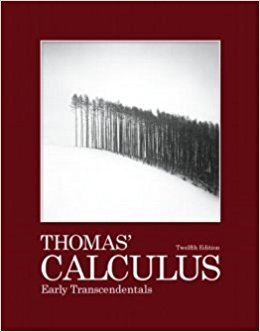×

×

# Solutions for Chapter 14: Partial Derivatives## Full solutions for Thomas' Calculus Early Transcendentals | 12th Edition

ISBN: 9780321588760Solutions for Chapter 14: Partial Derivatives

Solutions for Chapter 14
4 5 0 426 Reviews
31
4
##### ISBN: 9780321588760

Chapter 14: Partial Derivatives includes 102 full step-by-step solutions. Since 102 problems in chapter 14: Partial Derivatives have been answered, more than 33025 students have viewed full step-by-step solutions from this chapter. This textbook survival guide was created for the textbook: Thomas' Calculus Early Transcendentals, edition: 12. Thomas' Calculus Early Transcendentals was written by and is associated to the ISBN: 9780321588760. This expansive textbook survival guide covers the following chapters and their solutions.

Key Calculus Terms and definitions covered in this textbook
• Angle between vectors

The angle formed by two nonzero vectors sharing a common initial point

• Bounded below

A function is bounded below if there is a number b such that b ? ƒ(x) for all x in the domain of f.

• Cofunction identity

An identity that relates the sine, secant, or tangent to the cosine, cosecant, or cotangent, respectively

• Constant of variation

See Power function.

• Even function

A function whose graph is symmetric about the y-axis for all x in the domain of ƒ.

• Focus, foci

See Ellipse, Hyperbola, Parabola.

• Limit

limx:aƒ1x2 = L means that ƒ(x) gets arbitrarily close to L as x gets arbitrarily close (but not equal) to a

• Logarithm

An expression of the form logb x (see Logarithmic function)

• Modulus

See Absolute value of a complex number.

• Monomial function

A polynomial with exactly one term.

• Natural exponential function

The function ƒ1x2 = ex.

• Natural numbers

The numbers 1, 2, 3, . . . ,.

• Open interval

An interval that does not include its endpoints.

• Randomization

The principle of experimental design that makes it possible to use the laws of probability when making inferences.

• Rational numbers

Numbers that can be written as a/b, where a and b are integers, and b ? 0.

• Speed

The magnitude of the velocity vector, given by distance/time.

• Sum of an infinite series

See Convergence of a series

• Transpose of a matrix

The matrix AT obtained by interchanging the rows and columns of A.

• Velocity

A vector that specifies the motion of an object in terms of its speed and direction.

• Vertical asymptote

The line x = a is a vertical asymptote of the graph of the function ƒ if limx:a+ ƒ1x2 = q or lim x:a- ƒ1x2 = q.